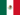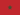ACT Sample Questions Part 2The New Alison App has just launched### This Course has been revised!

For a more enjoyable learning experience, we recommend that you study the mobile-friendly republished version of this course.

Take me to revised course.

- or -

Continue studying this course

# ACT Sample Questions Part 2

• Study Reminders

We'll email you at these times to remind you to study

You can set up to 7 reminders per week

#### You're all set

We'll email you at these times to remind you to study

Monday

Tuesday

Wednesday

Thursday

Friday

Saturday

Sunday

• Study Notes
• Discuss This TopicPetra S. 0 0 I never knew how to do ratio between a circle and a square. Helpful to now know the formula. One side of square is x and r is the radius of the circle. Formula to get r squared is x/2 squared plus x/2 squared. Figure out r. Square area equals length times width and circle equals Pi times r squared. Divide area of square by area of circle and the answer is 2/Pi.Jorge Carlos V. 0 0 There is a simpler solution: The square is two triangles with base 2r and height r, that is 2*squared(r). The area of circle is pi*squared(r).The requested ratio is the square area divided by the circle area, that is: 2/piKhalid B. 1 0 5/2πKhalid B. 0 0 3Khalid B. 0 0 1Khalid B. 0 0 0.25Khalid B. 0 0 0.50Linda E. 0 0 It was very helpfulCandance S. 0 0 I guess I was the only one who was lost by that whole equationHafid A. 0 0 G